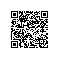# AOV网络及拓扑排序

/*       AOV网络及拓扑排序
1、在有向无环图中，用顶点表示活动，用有向边<u，v>表示活动u必须先与活动v,这种有向图叫AOV网络。
2、若<u，v>，则u是v的直接前驱，v是u的直接后继；若<u，u1，u2，···un，v>则称u是v的前驱，v是u的后继。
3、前驱后继关系有传递性和反自反性。则可以推断AOV网络必须是有向无环图。
4、拓扑排序实现方法：
1）从AOV网络中选择一个入度为0的顶点并输出；
2）从AOV网络中删除该顶点以及该顶点发出的所有边；
3）重复1）和2），直到找不到入度为0的顶点；
4）如果所有顶点都输出了则拓扑排序完成，否则说明此图是有环图。

顶点序号从1开始，输入文件最后一行为0 0 表示结束。

6 8                5 1 4 3 2 6
1 2                Network has a cycle!
1 4
2 6
3 2
3 6
5 1
5 2
5 6
6 8
1 3
1 2
2 5
3 4
4 2
4 6
5 4
5 6
0 0
*/
#include<stdio.h>
#include<string.h>
#define MAXN 10
struct Arcnode
{
int to;
struct Arcnode *next;
};
Arcnode* List[MAXN];
int n,m,count[MAXN];
char output;
void topsort()
{
int i,top=-1;
Arcnode* temp;
bool bcycle=false;
int pos=0;
for(i=0; i<n; i++)
if(count[i]==0)
{
count[i]=top;
top=i;
}
for(i=0; i<n; i++)
{
if(top==-1)
{
bcycle=true;
break;
}
else
{
int j=top;
top=count[top];
pos+=sprintf(output+pos,"%d ",j+1);
temp=List[j];
while(temp!=NULL)
{
int k=temp->to;
if(--count[k]==0)
{
count[k]=top;
top=k;
}
temp=temp->next;
}
}
}
if(bcycle)
printf("Network has a cycle!\n");
else
{
int len=strlen(output);
output[len-1]=0;
printf("%s\n",output);
}
}
int main()
{
int i,v,u;
//freopen("input.txt","r",stdin);
while(scanf("%d%d",&n,&m)!=EOF)
{
if(n==0 && m==0)
break;
memset(List,0,sizeof(List));
memset(count,0,sizeof(count));
memset(output,0,sizeof(output));
Arcnode* temp;
for(i=0;i<m;i++)
{
scanf("%d%d",&u,&v);
u--; v--;
count[v]++;
temp=new Arcnode;
temp->to=v;  temp->next=NULL;
if(List[u]==NULL)
List[u]=temp;
else
{
temp->next=List[u];
List[u]=temp;
}
}
topsort();
for(i=0;i<n;i++)
{
temp=List[i];
while(temp!=NULL)
{
List[i]=temp->next;
delete temp;
temp=List[i];
}
}
}
return 0;
}使用钉钉扫一扫加入圈子
+ 订阅